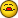# To combine various cell formulae in to the final cell

G

#### Gina G

Hi Group,

I have developed a sheet which has some cells for data entry and the
information is passed through several other cells with formula to massage
it to it's final form to a single cell for a result

C5: Enter data value "Degrees" as whole number for Site 1
D5: Enter data value "Minutes" as whole Number for site 1
C6: Enter data value "Degrees" as whole number for Site 1
D6; Enter data value "Minutes" as whole Number for site 1
H5: Enter data value "Degrees" as whole number for Site 1
I5: Enter data value "Minutes" as whole Number for site 1
H6: Enter data value "Degrees" as whole number for Site 1
I6: Enter data value "Minutes" as whole Number for Site 1
B1: Select area name from dropdown list (P25)
G1: Select area name from dropdown list (P25)

C1: =LOOKUP(B1,P25,Q2:Q5)
D1: =LOOKUP(B1,P25,R2:R5)

H1: =LOOKUP(G1,P25,Q2:Q5)
I1: =LOOKUP(G1,P25,R2:R5)

E5: =(D5/60+C5)*C1
E6: =(D6/60+C6)*D1

J5: =(I5/60+H5)*H1
J6: =(I6/60+H6)*I1

L5: =J5-E5
L6: =J6-E6

M5: =ABS(E5-J5)
M6: =ABS(E6-J6)

N5: =IF(L5>0,1,-1)
N6: =IF(L6>0,1,-1)
N7: =N5+N6*2

Q25: =L6 Result used seperately later
R25:

Q29: =AND(Q25>-180,Q25<180)

R28: =IF(Q25=180,180,0)
R29: =Q25*Q29
R30: =IF(Q25<-180,360-Q25,0)
R31: =IF(Q25>180,360-Q25,0)

S28: =IF(R28=0,0,1)
S29: =IF(R29=0,0,1)
S30: =IF(R30=0,0,1)
S31: =IF(R31=0,0,1)

Cells S28:,S29: S30:, AND S31: are then used as switches to further

Below I have manual rebuilt S31 from the information above. I want the
detail how to get Excel to build the code automatically.
= = = = = = =
S31: =IF(=IF((=(I6/60+H6)*(=LOOKUP(G1,P25,R2:R5))-(=(D6/60+C6)*D1))
180,360-(=(I6/60+H6)*(=LOOKUP(G1,P25,R2:R5))-(=(D6/60+C6)*D1)),0)
=0,0,1)
= = = = = =
I want to make a single line of 'code' for the result cells by combining
the functions that feed into them. As an examlpe of what I mean, I have
manually built the 'code for S35: as an example.

D

#### David

Gina
Difficult to track what each element is doing without examples- if you like
send a sample of spreadsheet with sample data to (e-mail address removed) and I'll
have a look at it for you